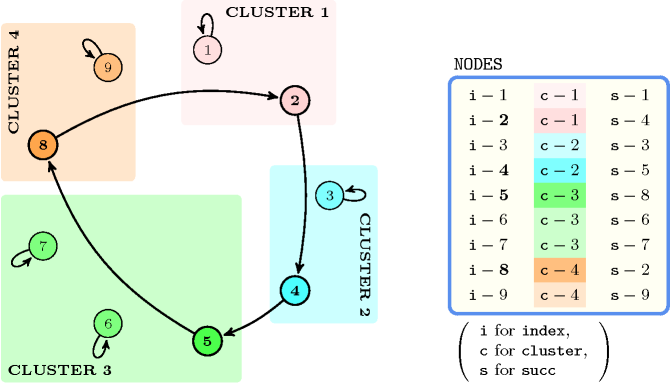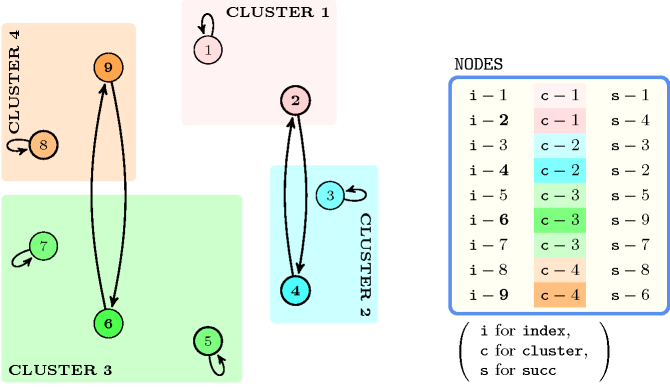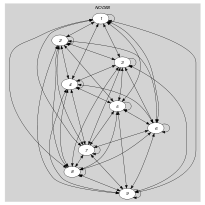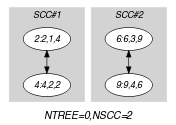## 5.67. circuit_cluster

Origin

Inspired by [LaporteAsefVaziriSriskandarajah96].

Constraint

$\mathrm{𝚌𝚒𝚛𝚌𝚞𝚒𝚝}_\mathrm{𝚌𝚕𝚞𝚜𝚝𝚎𝚛}\left(\mathrm{𝙽𝙲𝙸𝚁𝙲𝚄𝙸𝚃},\mathrm{𝙽𝙾𝙳𝙴𝚂}\right)$

Arguments
 $\mathrm{𝙽𝙲𝙸𝚁𝙲𝚄𝙸𝚃}$ $\mathrm{𝚍𝚟𝚊𝚛}$ $\mathrm{𝙽𝙾𝙳𝙴𝚂}$ $\mathrm{𝚌𝚘𝚕𝚕𝚎𝚌𝚝𝚒𝚘𝚗}\left(\mathrm{𝚒𝚗𝚍𝚎𝚡}-\mathrm{𝚒𝚗𝚝},\mathrm{𝚌𝚕𝚞𝚜𝚝𝚎𝚛}-\mathrm{𝚒𝚗𝚝},\mathrm{𝚜𝚞𝚌𝚌}-\mathrm{𝚍𝚟𝚊𝚛}\right)$
Restrictions
 $\mathrm{𝙽𝙲𝙸𝚁𝙲𝚄𝙸𝚃}\ge 1$ $\mathrm{𝙽𝙲𝙸𝚁𝙲𝚄𝙸𝚃}\le |\mathrm{𝙽𝙾𝙳𝙴𝚂}|$ $\mathrm{𝚛𝚎𝚚𝚞𝚒𝚛𝚎𝚍}$$\left(\mathrm{𝙽𝙾𝙳𝙴𝚂},\left[\mathrm{𝚒𝚗𝚍𝚎𝚡},\mathrm{𝚌𝚕𝚞𝚜𝚝𝚎𝚛},\mathrm{𝚜𝚞𝚌𝚌}\right]\right)$ $\mathrm{𝙽𝙾𝙳𝙴𝚂}.\mathrm{𝚒𝚗𝚍𝚎𝚡}\ge 1$ $\mathrm{𝙽𝙾𝙳𝙴𝚂}.\mathrm{𝚒𝚗𝚍𝚎𝚡}\le |\mathrm{𝙽𝙾𝙳𝙴𝚂}|$ $\mathrm{𝚍𝚒𝚜𝚝𝚒𝚗𝚌𝚝}$$\left(\mathrm{𝙽𝙾𝙳𝙴𝚂},\mathrm{𝚒𝚗𝚍𝚎𝚡}\right)$ $\mathrm{𝙽𝙾𝙳𝙴𝚂}.\mathrm{𝚜𝚞𝚌𝚌}\ge 1$ $\mathrm{𝙽𝙾𝙳𝙴𝚂}.\mathrm{𝚜𝚞𝚌𝚌}\le |\mathrm{𝙽𝙾𝙳𝙴𝚂}|$
Purpose

Consider a digraph $G$, described by the $\mathrm{𝙽𝙾𝙳𝙴𝚂}$ collection, such that its vertices are partitioned among several clusters. $\mathrm{𝙽𝙲𝙸𝚁𝙲𝚄𝙸𝚃}$ is the number of circuits containing more than one vertex used for covering $G$ in such a way that each cluster is visited by exactly one circuit of length greater than 1.

Example
 $\left(\begin{array}{c}1,〈\begin{array}{ccc}\mathrm{𝚒𝚗𝚍𝚎𝚡}-1\hfill & \mathrm{𝚌𝚕𝚞𝚜𝚝𝚎𝚛}-1\hfill & \mathrm{𝚜𝚞𝚌𝚌}-1,\hfill \\ \mathrm{𝚒𝚗𝚍𝚎𝚡}-2\hfill & \mathrm{𝚌𝚕𝚞𝚜𝚝𝚎𝚛}-1\hfill & \mathrm{𝚜𝚞𝚌𝚌}-4,\hfill \\ \mathrm{𝚒𝚗𝚍𝚎𝚡}-3\hfill & \mathrm{𝚌𝚕𝚞𝚜𝚝𝚎𝚛}-2\hfill & \mathrm{𝚜𝚞𝚌𝚌}-3,\hfill \\ \mathrm{𝚒𝚗𝚍𝚎𝚡}-4\hfill & \mathrm{𝚌𝚕𝚞𝚜𝚝𝚎𝚛}-2\hfill & \mathrm{𝚜𝚞𝚌𝚌}-5,\hfill \\ \mathrm{𝚒𝚗𝚍𝚎𝚡}-5\hfill & \mathrm{𝚌𝚕𝚞𝚜𝚝𝚎𝚛}-3\hfill & \mathrm{𝚜𝚞𝚌𝚌}-8,\hfill \\ \mathrm{𝚒𝚗𝚍𝚎𝚡}-6\hfill & \mathrm{𝚌𝚕𝚞𝚜𝚝𝚎𝚛}-3\hfill & \mathrm{𝚜𝚞𝚌𝚌}-6,\hfill \\ \mathrm{𝚒𝚗𝚍𝚎𝚡}-7\hfill & \mathrm{𝚌𝚕𝚞𝚜𝚝𝚎𝚛}-3\hfill & \mathrm{𝚜𝚞𝚌𝚌}-7,\hfill \\ \mathrm{𝚒𝚗𝚍𝚎𝚡}-8\hfill & \mathrm{𝚌𝚕𝚞𝚜𝚝𝚎𝚛}-4\hfill & \mathrm{𝚜𝚞𝚌𝚌}-2,\hfill \\ \mathrm{𝚒𝚗𝚍𝚎𝚡}-9\hfill & \mathrm{𝚌𝚕𝚞𝚜𝚝𝚎𝚛}-4\hfill & \mathrm{𝚜𝚞𝚌𝚌}-9\hfill \end{array}〉\hfill \end{array}\right)$ $\left(\begin{array}{c}2,〈\begin{array}{ccc}\mathrm{𝚒𝚗𝚍𝚎𝚡}-1\hfill & \mathrm{𝚌𝚕𝚞𝚜𝚝𝚎𝚛}-1\hfill & \mathrm{𝚜𝚞𝚌𝚌}-1,\hfill \\ \mathrm{𝚒𝚗𝚍𝚎𝚡}-2\hfill & \mathrm{𝚌𝚕𝚞𝚜𝚝𝚎𝚛}-1\hfill & \mathrm{𝚜𝚞𝚌𝚌}-4,\hfill \\ \mathrm{𝚒𝚗𝚍𝚎𝚡}-3\hfill & \mathrm{𝚌𝚕𝚞𝚜𝚝𝚎𝚛}-2\hfill & \mathrm{𝚜𝚞𝚌𝚌}-3,\hfill \\ \mathrm{𝚒𝚗𝚍𝚎𝚡}-4\hfill & \mathrm{𝚌𝚕𝚞𝚜𝚝𝚎𝚛}-2\hfill & \mathrm{𝚜𝚞𝚌𝚌}-2,\hfill \\ \mathrm{𝚒𝚗𝚍𝚎𝚡}-5\hfill & \mathrm{𝚌𝚕𝚞𝚜𝚝𝚎𝚛}-3\hfill & \mathrm{𝚜𝚞𝚌𝚌}-5,\hfill \\ \mathrm{𝚒𝚗𝚍𝚎𝚡}-6\hfill & \mathrm{𝚌𝚕𝚞𝚜𝚝𝚎𝚛}-3\hfill & \mathrm{𝚜𝚞𝚌𝚌}-9,\hfill \\ \mathrm{𝚒𝚗𝚍𝚎𝚡}-7\hfill & \mathrm{𝚌𝚕𝚞𝚜𝚝𝚎𝚛}-3\hfill & \mathrm{𝚜𝚞𝚌𝚌}-7,\hfill \\ \mathrm{𝚒𝚗𝚍𝚎𝚡}-8\hfill & \mathrm{𝚌𝚕𝚞𝚜𝚝𝚎𝚛}-4\hfill & \mathrm{𝚜𝚞𝚌𝚌}-8,\hfill \\ \mathrm{𝚒𝚗𝚍𝚎𝚡}-9\hfill & \mathrm{𝚌𝚕𝚞𝚜𝚝𝚎𝚛}-4\hfill & \mathrm{𝚜𝚞𝚌𝚌}-6\hfill \end{array}〉\hfill \end{array}\right)$
##### Figure 5.67.1. Four clusters and a covering with one circuit corresponding to the first example of the Example slotBoth examples involve 9 vertices $1,2,\cdots ,9$ such that vertices 1 and 2 belong to cluster number 1, vertices 3 and 4 belong to cluster number 2, vertices 5, 6 and 7 belong to cluster number 3, and vertices 8 and 9 belong to cluster number 4.

The first example involves only a single circuit containing more than one vertex (i.e., see in Figure 5.67.1 the circuit $2\to 4\to 5\to 8\to 2$). The corresponding $\mathrm{𝚌𝚒𝚛𝚌𝚞𝚒𝚝}_\mathrm{𝚌𝚕𝚞𝚜𝚝𝚎𝚛}$ constraint holds since exactly one vertex of each cluster (i.e., vertex 2 for cluster 1, vertex 4 for cluster 2, vertex 5 for cluster 3, vertex 8 for cluster 4) belongs to this circuit.

The second example contains the two circuits $2\to 4\to 2$ and $6\to 9\to 6$ that both involve more than one vertex. The corresponding $\mathrm{𝚌𝚒𝚛𝚌𝚞𝚒𝚝}_\mathrm{𝚌𝚕𝚞𝚜𝚝𝚎𝚛}$ constraint holds since exactly one vertex of each cluster (i.e., see in Figure 5.67.2 vertex 2 in $2\to 4\to 2$ for cluster 1, vertex 4 in $2\to 4\to 2$ for cluster 2, vertex 6 in $6\to 9\to 6$ for cluster 3, vertex 9 in $6\to 9\to 6$ for cluster 4) belongs to these two circuits.

##### Figure 5.67.2. The same clusters as in the first example of the Example slot and a covering with two circuits corresponding to the second example of the Example slotTypical
 $\mathrm{𝙽𝙲𝙸𝚁𝙲𝚄𝙸𝚃}<|\mathrm{𝙽𝙾𝙳𝙴𝚂}|$ $|\mathrm{𝙽𝙾𝙳𝙴𝚂}|>2$ $\mathrm{𝚛𝚊𝚗𝚐𝚎}$$\left(\mathrm{𝙽𝙾𝙳𝙴𝚂}.\mathrm{𝚌𝚕𝚞𝚜𝚝𝚎𝚛}\right)>1$
Symmetry

Items of $\mathrm{𝙽𝙾𝙳𝙴𝚂}$ are permutable.

Usage

A related abstraction in Operations Research was introduced in [LaporteAsefVaziriSriskandarajah96]. It was reported as the Generalised Travelling Salesman Problem (GTSP). The $\mathrm{𝚌𝚒𝚛𝚌𝚞𝚒𝚝}_\mathrm{𝚌𝚕𝚞𝚜𝚝𝚎𝚛}$ constraint differs from the GTSP because of the two following points:

• Each node of our graph belongs to a single cluster,

• We do not constrain the number of circuits to be equal to 1: The number of circuits should be equal to one of the values of the domain of the variable $\mathrm{𝙽𝙲𝙸𝚁𝙲𝚄𝙸𝚃}$.

Keywords
Arc input(s)

$\mathrm{𝙽𝙾𝙳𝙴𝚂}$

Arc generator
$\mathrm{𝐶𝐿𝐼𝑄𝑈𝐸}$$↦\mathrm{𝚌𝚘𝚕𝚕𝚎𝚌𝚝𝚒𝚘𝚗}\left(\mathrm{𝚗𝚘𝚍𝚎𝚜}\mathtt{1},\mathrm{𝚗𝚘𝚍𝚎𝚜}\mathtt{2}\right)$

Arc arity
Arc constraint(s)
 $•\mathrm{𝚗𝚘𝚍𝚎𝚜}\mathtt{1}.\mathrm{𝚜𝚞𝚌𝚌}\ne \mathrm{𝚗𝚘𝚍𝚎𝚜}\mathtt{1}.\mathrm{𝚒𝚗𝚍𝚎𝚡}$ $•\mathrm{𝚗𝚘𝚍𝚎𝚜}\mathtt{1}.\mathrm{𝚜𝚞𝚌𝚌}=\mathrm{𝚗𝚘𝚍𝚎𝚜}\mathtt{2}.\mathrm{𝚒𝚗𝚍𝚎𝚡}$
Graph property(ies)
 $•$$\mathrm{𝐍𝐓𝐑𝐄𝐄}$$=0$ $•$$\mathrm{𝐍𝐒𝐂𝐂}$$=\mathrm{𝙽𝙲𝙸𝚁𝙲𝚄𝙸𝚃}$

Graph class
$\mathrm{𝙾𝙽𝙴}_\mathrm{𝚂𝚄𝙲𝙲}$

Sets
$\begin{array}{c}\mathrm{𝖠𝖫𝖫}_\mathrm{𝖵𝖤𝖱𝖳𝖨𝖢𝖤𝖲}↦\hfill \\ \left[\begin{array}{c}\mathrm{𝚟𝚊𝚛𝚒𝚊𝚋𝚕𝚎𝚜}-\mathrm{𝚌𝚘𝚕}\left(\begin{array}{c}\mathrm{𝚅𝙰𝚁𝙸𝙰𝙱𝙻𝙴𝚂}-\mathrm{𝚌𝚘𝚕𝚕𝚎𝚌𝚝𝚒𝚘𝚗}\left(\mathrm{𝚟𝚊𝚛}-\mathrm{𝚍𝚟𝚊𝚛}\right),\hfill \\ \mathrm{𝚒𝚝𝚎𝚖}\left(\mathrm{𝚟𝚊𝚛}-\mathrm{𝙽𝙾𝙳𝙴𝚂}.\mathrm{𝚌𝚕𝚞𝚜𝚝𝚎𝚛}\right)\right]\hfill \end{array}\right)\hfill \end{array}\right]\hfill \end{array}$

Constraint(s) on sets
 $•$$\mathrm{𝚊𝚕𝚕𝚍𝚒𝚏𝚏𝚎𝚛𝚎𝚗𝚝}$$\left(\mathrm{𝚟𝚊𝚛𝚒𝚊𝚋𝚕𝚎𝚜}\right)$ $•$$\mathrm{𝚗𝚟𝚊𝚕𝚞𝚎𝚜}$$\left(\mathrm{𝚟𝚊𝚛𝚒𝚊𝚋𝚕𝚎𝚜},=,\mathrm{𝚜𝚒𝚣𝚎}\left(\mathrm{𝙽𝙾𝙳𝙴𝚂},\mathrm{𝚌𝚕𝚞𝚜𝚝𝚎𝚛}\right)\right)$
Graph model

In order to express the binary constraint linking two vertices one has to make explicit the identifier of each vertex as well as the cluster to which belongs each vertex. This is why the $\mathrm{𝚌𝚒𝚛𝚌𝚞𝚒𝚝}_\mathrm{𝚌𝚕𝚞𝚜𝚝𝚎𝚛}$ constraint considers objects that have the following three attributes:

• The attribute $\mathrm{𝚒𝚗𝚍𝚎𝚡}$ that is the identifier of a vertex.

• The attribute $\mathrm{𝚌𝚕𝚞𝚜𝚝𝚎𝚛}$ that is the cluster to which belongs a vertex.

• The attribute $\mathrm{𝚜𝚞𝚌𝚌}$ that is the unique successor of a vertex.

The partitioning of the clusters by different circuits is expressed in the following way:

Parts (A) and (B) of Figure 5.67.3 respectively show the initial and final graph associated with the second example of the Example slot. Since we use the $\mathrm{𝐍𝐒𝐂𝐂}$ graph property, we show the two strongly connected components of the final graph. They respectively correspond to the two circuits $2\to 4\to 2$ and $6\to 9\to 6$. Since all the vertices belongs to a circuit we have that $\mathrm{𝐍𝐓𝐑𝐄𝐄}$ $=$ 0.

##### Figure 5.67.3. Initial and final graph of the $\mathrm{𝚌𝚒𝚛𝚌𝚞𝚒𝚝}_\mathrm{𝚌𝚕𝚞𝚜𝚝𝚎𝚛}$ constraint(a) (b)